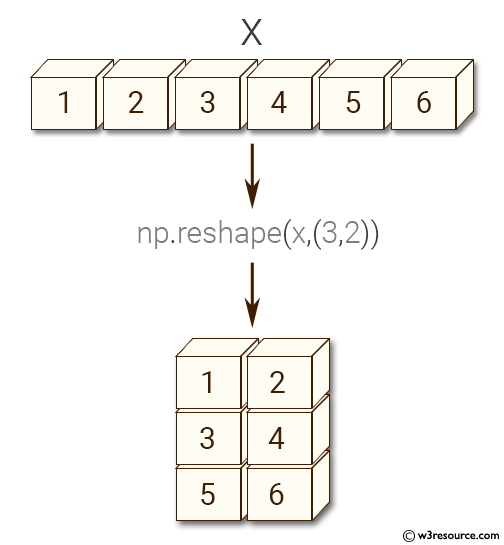﻿ NumPy: Create a new shape to an array without changing its data - w3resource# NumPy: Create a new shape to an array without changing its data

## NumPy: Array Object Exercise-38 with Solution

Write a NumPy program to create a new shape to an array without changing its data.

Pictorial Presentation:Sample Solution:-

Python Code:

``````import numpy as np
x = np.array([1, 2, 3, 4, 5, 6])
y = np.reshape(x,(3,2))
print("Reshape 3x2:")
print(y)
z = np.reshape(x,(2,3))
print("Reshape 2x3:")
print(z)
```
```

Sample Output:

```Reshape 3x2:
[[1 2]
[3 4]
[5 6]]
Reshape 2x3:
[[1 2 3]
[4 5 6]]
```

Python Code Editor:

Have another way to solve this solution? Contribute your code (and comments) through Disqus.

What is the difficulty level of this exercise?

Test your Python skills with w3resource's quiz

﻿

## Python: Tips of the Day

Set comprehension:

```>>> m = {x ** 2 for x in range(5)}
>>> m
{0, 1, 4, 9, 16}
```